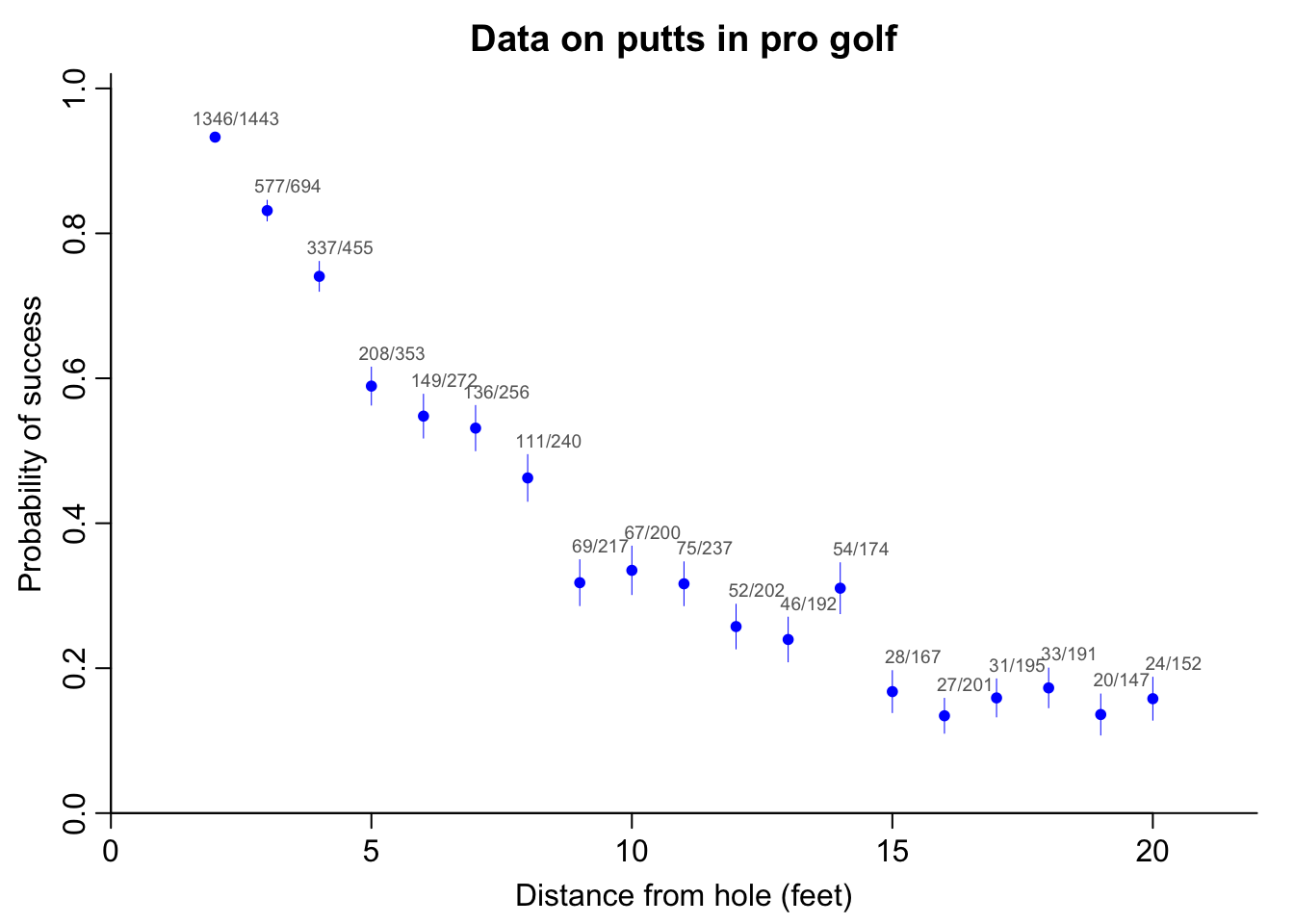The following graph shows data from professional golfers on the proportion of successful putts as a function of distance from the hole. Unsurprisingly, the probability of making the shot declines as a function of distance:The error bars associated with each point $$j$$ in the above graph are simple classical standard deviations, $$\sqrt{\hat{p}_j(1-\hat{p}_j)/n_j}$$, where $$\hat{p}_j=y_j/n_j$$ is the success rate for putts taken at distance $$x_j$$.

### Logistic regression

Can we model the probability of success in golf putting as a function of distance from the hole? Given usual statistical practice, the natural starting point would be logistic regression:

$y_j\sim\mbox{binomial}(n_j, \mbox{logit}^{-1}(a + bx_j)), \mbox{ for } j=1,\dots, J.$ In Stan, this is:

data {
int J;
int n[J];
vector[J] x;
int y[J];
}
parameters {
real a;
real b;
}
model {
y ~ binomial_logit(n, a + b*x);
}

The code in the above model block is (implicitly) vectorized, so that it is mathematically equivalent to modeling each data point, y[i] ~ binomial_logit(n[i], a + b*x[i]). The vectorized code is more compact (no need to write a loop, or to include the subscripts) and faster (because of more efficient gradient evaluations).

We fit the model to the data:

golf_data <- list(x=x, y=y, n=n, J=J)
fit_logistic <- stan("golf_logistic.stan", data=golf_data)
a_sim <- extract(fit_logistic)$a b_sim <- extract(fit_logistic)$b

And here is the result:

Inference for Stan model: golf_logistic.
4 chains, each with iter=2000; warmup=1000; thin=1;
post-warmup draws per chain=1000, total post-warmup draws=4000.

mean se_mean   sd   25%   50%   75% n_eff Rhat
a  2.23       0 0.06  2.19  2.23  2.27  1157    1
b -0.26       0 0.01 -0.26 -0.26 -0.25  1170    1

Samples were drawn using NUTS(diag_e) at Tue Oct  1 15:56:33 2019.
For each parameter, n_eff is a crude measure of effective sample size,
and Rhat is the potential scale reduction factor on split chains (at
convergence, Rhat=1).

Going through the columns of the above table: Stan has computed the posterior means $$\pm$$ standard deviations of $$a$$ and $$b$$ to be 2.23 $$\pm$$ 0.06 and -0.26 $$\pm$$ 0.01, respectively. The Monte Carlo standard error of the mean of each of these parameters is 0 (to two decimal places), indicating that the simulations have run long enough to estimate the posterior means precisely. The posterior quantiles give a sense of the uncertainty in the parameters, with 50% posterior intervals of [2.19, 2.27] and [-0.26, -0.25] for $$a$$ and $$b$$, respectively. Finally, the values of $$\widehat{R}$$ near 1 tell us that the simulations from Stan’s four simulated chains have mixed well. (We have more sophisticated convergence diagnostics, and also we recommend checking the fit using simulated data, as discussed in the Bayesian Workflow Using Stan book, but checking that $$\widehat{R}$$ is near 1 is a good start.)

The following graph shows the fit plotted along with the data: